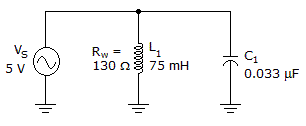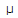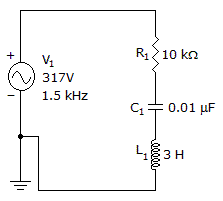# Electronics - RLC Circuits and Resonance

### Exercise :: RLC Circuits and Resonance - General Questions

31.

What is the total impedance of a 60 Hz series RLC circuit when XL = 7.5 ohms, XC = 265.3 ohms, and R = 33 ohms?

 A. 257.8 ohms B. 259.9 ohms C. 290.8 ohms D. 1989.75 ohms

Answer: Option B

Explanation:

No answer description available for this question. Let us discuss.

32.What is the total current for the nonideal circuit in the given circuit. If it is at resonance?

 A. 0 A B. 284A C. 3.3 mA D. 38.5 mA

Answer: Option B

Explanation:

No answer description available for this question. Let us discuss.

33.

What is the high cutoff frequency for an RLC circuit that resonates at 2000 Hz and has a bandwidth of 250 Hz?

 A. 2125 Hz B. 2250 Hz C. 1750 Hz D. 8.0 Hz

Answer: Option A

Explanation:

No answer description available for this question. Let us discuss.

34.

Current in a series RLC circuit may always be used as:

 A. a leading vector B. a reference C. an angle D. a lagging vector

Answer: Option B

Explanation:

No answer description available for this question. Let us discuss.

35.

What is the impedance of the circuit?A. 12.2 kB. 14.5 kC. 17.8 kD. 20.3 kAnswer: Option D

Explanation:

No answer description available for this question. Let us discuss.

#### Current Affairs 2021

Interview Questions and Answers